The Imperial System

Imperial Units of Measure

 Length Weight Capacity 1 ft = 12 in. 1 lb = 16 oz 1 c = 8 fl oz 1 yd = 3 ft = 36 in. 1 T = 2000 lb 1 pt = 2 c 1 mi = 1760 yd = 5280 ft 1 qt = 2 pt 1 gal = 4 qt

Helpful hint: To convert units, multiply by a convenient form of 1.

For example, to convert inches to feet, multiply by .

Converting Imperial Units of Length

Example

In 1976, the artist Christo built a 24 1/2 mile long fabric fence in California.  He and his team joined pieces of fabric, each 63 feet long, together to form the fence.  How many yards long was each piece?

Solution

63 ft x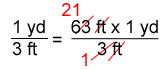Multiply the fractions.

= 21 yd                 Simplify the product.

Answer: Each piece of fabric was 21 yards long.

Converting Imperial Units of Weight

Example

A humpback whale weighs 33 tons.  How many pounds is this?

*Hint: 1 T = 2000 lb.*

Solution

33 T x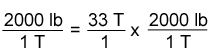Write 33 T as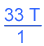.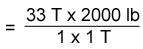Cancel out the unit "T".

Multiply the numbers in the numerator.

Divide.

= 66,000 lb

Answer:  The humpback whale weighs 66,000 pounds.

Converting Customary Units of Capacity

Example

Convert 25 fluid ounces to pints.

Use the fact that 1 c = 8 fl oz and 1 pt = 2 c.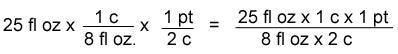Use rule for multiplying fractions.  Divide out common units.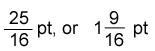Multiply.

Answer:  25 fluid ounces is equal to 1 9/16 pints.

Practice

Complete each statement with feetinches, or yards.

1.   1 mi = 1760 ___________

2.   1 mi = 63,360 ___________

3.   1 mi = 5280 ___________

Complete each statement.

4.    32 oz = ___________ lb

5.    17 pt = ___________ c

6.    41 in. = ___________ ft

Writing Measurements in Mixed Units.

Example

Convert 26 fluid ounces to cups and fluid ounces.

Step 1: Convert 26 fluid ounces to cups.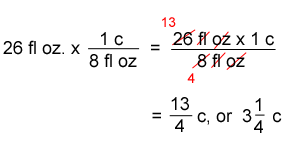Step 2: Convert the fractional part from cups to fluid ounces.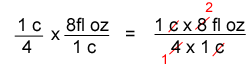= 2 fl oz

Answer: 26 fluid ounces = 3 cups 2 fluid ounces.

Adding and Subtracting with Mixed Units.

Example

Your school backpack weighs 7 pounds 6 ounces.  Your younger brother's backpack weighs 6 pounds 14 ounces.

a. Find the sum of the weights.

7 lb   6 oz
+ 6 lb 14 oz
13 lb 20 oz

We can rename the sum since there are 16 ounces in a pound.

13 lb 20 oz = 13 lb (1 lb 4 oz) = 14 lb 4 oz

Answer:  The sum is 14 lb 4 oz.

b.  Find the difference of the weights.

7 lb   6 oz
- 6 lb 14 oz

Since we cannot take 14 away from 6, we need to rename.

7 lb 6 oz = (6 lb 16 oz) 6 oz = 6 lb 22 oz

6 lb 22 oz
- 6 lb 16 oz
6 oz

Answer: The difference is 6 oz.

Add and write in simplest form.

7.      5 c   7 fl oz  +   7 c    8 fl oz

8.    9 lb   3 oz  +  4 lb   15 oz

Subtract and write in simplest form.

9.   12 ft  2 in. -  7 ft  10 in.

10.  One lap of the Indy 500 is 2 1/2 miles long. How many feet are in three complete laps?

A. 1250 feet
B. 13,200 feet
C. 39,600 feet
D. 79,200 feet

11.   Sally has 4 gallons of juice.  Could she serve 75 people each 1 cup?  How much more will she need or have left over?

Complete each statement.

12.   lb = __________ oz

13.  T = __________ lb

14.  c = __________ fl oz

15. 103 in. = __________ yd __________ ft

16. 9 qt = __________ gal __________ qt

17. 78 oz = __________ lb __________ oz

Math challenge!

Find the weight of each loaf of bread.

• The rye bread weighs 1 lb 7 oz more than the wheat bread.
• The corn bread weighs half as much as the wheat bread.
• The wheat bread weighs 6 oz less than 2 pounds.

1.  yards

2.  inches

3.  feet

4.  2

5.  34

6.  3 5/12

7.  13 c  7 fl oz

8.  14 lb  2 oz

9.  4 ft  4 in

10.  C

11.  No, she needs 11 more cups.

12.  74

13.  2500

14.  30

15.  2 yd  2 7/12 ft  (or 2 yd  2 ft  7 in)

16.  2 gal  1 qt

17.  4 lb  14 oz

Challenge
Wheat bread:  1 lb  10 oz
Rye bread:  3 lb  1 oz